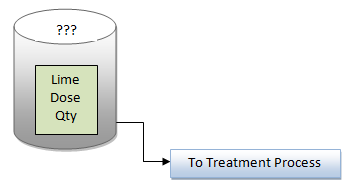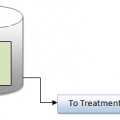## How to Calculate Lime Dosage Requirement in Sedimentation ProcessLime dosage requirement is most important process in Sedimentation Process, to separate the solids from liquid by gravity. Sedimentation is the basic process, whether water and wastewater treatment,

In water treatment process, first remove Girt / fine fibers in presedimentation basin and follows coagulation–flocculation is most commonly used.

We find adequate /require amount of alkalinity (HCO3) first,which will help to proper precipitation in alum dosage process.

three steps are required, to find necessary lime dose (in mg/L),

First, the total alkalinity required is calculated. The total alkalinity required to react with the
alum to be added and give proper precipitation is calculated using the this equation:

Total alkalinity required (mg/L) = Alkalinity reacting with alum (mg/L) + alkalinity presence in the water (mg/L)   {?1 mg/L alum reacts with 0.45 mg/L alkalinity}.

Second step, we make a comparison between required alkalinity and alkalinity already in
the raw water to determine how many mg/L alkalinity should be added to the water. Equation
is used to make this calculation:Alkalinity to be added to the water (mg/ L) = total alkalinity required (mg/ L) – (minus) alkalinity present in the water (mg/ L)

Finally, after determining the amount of alkalinity to be added to the water, we find how
much lime (the source of alkalinity) must be added. We accomplish this by using the ratio on below equation

Determine/calculate the mg/L lime required by using a proportion that relates bicarbonate alkalinity to lime:

0.45 mg / L alk         total alkalinity required mg/l alk.
———————-  =   —————————————
0.35 mg/ L lime       determine total Lime require mg/l

Using the afore said three steps, you can calculate/determine the lime dosage (mg/L) requirement to you process .## Author:T.P.Sivanandan

#### Why Fine Bubbles are Better than Coarse bubble in Aeration Tank#### How to Calculate Lime Dosage Requirement in Sedimentation Process